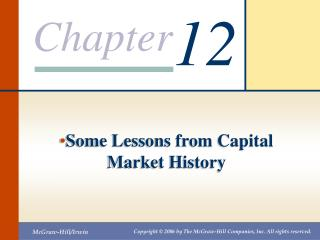DownloadDownload PresentationSome Lessons from Capital Market History

# Some Lessons from Capital Market History

Download Presentation## Some Lessons from Capital Market History

- - - - - - - - - - - - - - - - - - - - - - - - - - - E N D - - - - - - - - - - - - - - - - - - - - - - - - - - -
##### Presentation Transcript

1. 12 Some Lessons from Capital Market History

2. Chapter 12 – Index of Sample Problems • Slide # 02 - 03 Dividend yield • Slide # 04 - 05 Capital gains yield • Slide # 06 - 07 Total return • Slide # 08 - 09 Nominal vs. real returns • Slide # 10 - 11 Risk premium • Slide # 12 - 13 Average return • Slide # 14 - 15 Variance • Slide # 16 - 17 Standard deviation • Slide # 18 - 23 Probability distributions • Slide # 24 - 26 Arithmetic vs. geometric averages

3. 2: Dividend yield The common stock of Abaco Co. is expected to pay \$1.60 in dividends next year. Currently, the stock is selling for \$38.90 a share. What is the dividend yield?

4. 3: Dividend yield

5. 4: Capital gains yield Last year, you purchased shares of Baker and Sons, Inc. at a price of \$28.42 a share. Since that time you have received \$1.20 in dividends per share. Currently, the stock is selling for \$31.18 per share. What is the capital gains yield?

6. 5: Capital gains yield

7. 6: Total return Zoma Enterprises pays \$.80 a year as a dividend on their common stock. Currently, this stock sells for \$28.12 a share. Last year at this time the stock was selling for \$31.64 a share. What is the total return on this stock in dollars? What is the percentage total return?

8. 7: Total return

9. History of securities (p.367) • Large company • Small company • Long-term Government bond • Treasury bill • inflation

10. 8: Nominal vs. real returns Last year, you purchased shares of Benson and Judges, Inc. stock for \$13.50 a share. Since then you received \$.50 per share in dividends. Today, you sold your shares for \$18.20 a share. The inflation rate for the period is 3.5%. What is your nominal rate of return? What is your real rate of return?

11. 9: Nominal vs. real returns

12. 10: Risk premium Assume that the following are the average annual returns for the past decade: Large-company stocks 9.6% Long-term corporate bonds 5.8% U.S. Treasury bills 2.5% Inflation 1.9% What is the risk premium on large-company stocks for this time period?

14. 12: Average return A stock returned 4.8%, 9.3%, 21.6%, -13.2% and 0.4% for the past five years, respectively. What is the average rate of return for the past five years?

15. 13: Average return

16. 14: Variance A stock returned 4.8%, 9.3%, 21.6%, -13.2% and 0.4% for the past five years, respectively. What is the variance?

17. 15: Variance

18. 16: Standard deviation A stock returned 4.8%, 9.3%, 21.6%, -13.2% and 0.4% for the past five years, respectively. The variance is .016125. What is the standard deviation?

19. 17: Standard deviation The variance, 2, as computed previously, is .016125.

20. 18: Probability distributions A stock has an average rate of return of 4.58% and a standard deviation of 12.70%. Assume that the returns are normally distributed. What range of returns would you expect to see 68% of the time? 95% of the time? 99% of the time?

21. 19: Probability distributions

22. 20: Probability distributions

23. 21: Probability distributions

24. 22: Probability distributions A stock has an average rate of return of 12.9% and a standard deviation of 15.3%. Assume the returns are normally distributed. What is the probability that you will lose more than one-third of your investment in this stock in any one year?

25. 23: Probability distributions The probability of losing more than one-third (33%) of your investment in this stock in any one year is less than ½ of 1%.

26. 24: Arithmetic vs. geometric averages A stock has the following year-end prices and dividends. Year Price Dividend 0 \$38.16 --- 1 \$39.43 \$.60 2 \$38.04 \$.62 3 \$45.09 \$.65 4 \$44.10 \$.70 What are the arithmetic and geometric returns for this stock?

27. 25: Arithmetic vs. geometric averages

28. 26: Arithmetic vs. geometric averages Annual returns: 4.90%, -1.95%, 20.24% and -.64%

29. Arithmetic average: good for guess the return of one period: optimistic • Geometric average: good for guess the return of long term: pessimistic

30. Capital market efficiency • Degree of reflecting information • Efficiency Market Hypothesis (EMH) • Strong form: all available information • Semistrong form: all public information • Weak form: current price reflect all past stock’s price

31. 12 End of Chapter 12